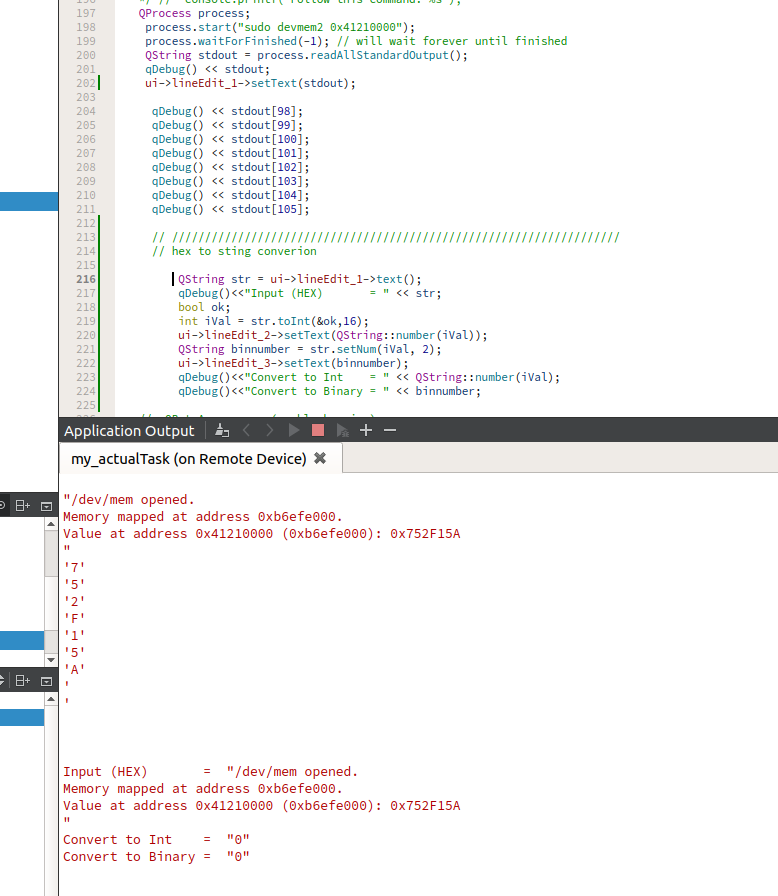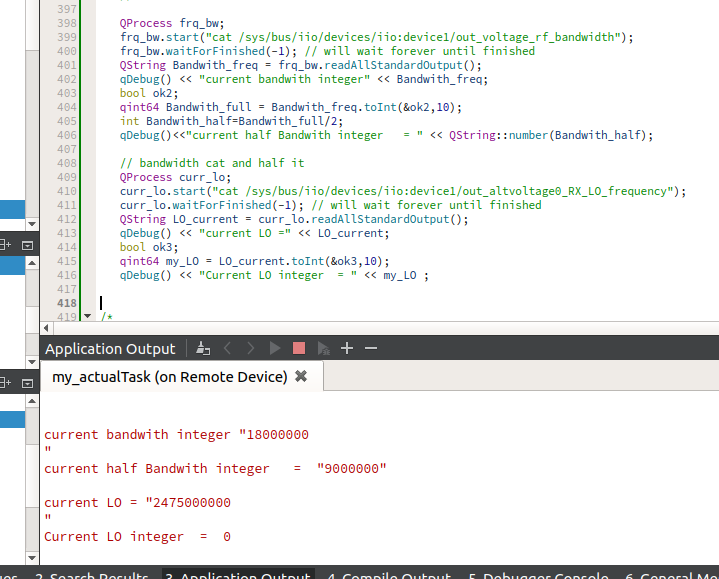# how to convert QCharRef to hex, int, binary

• I know how to convert hex into int, binary.

``````     QString str = ui->lineEdit_1->text();
qDebug()<<"Input (HEX)       = " << str;
bool ok;
int iVal = str.toInt(&ok,16);
ui->lineEdit_2->setText(QString::number(iVal));
QString binnumber = str.setNum(iVal, 2);
ui->lineEdit_3->setText(binnumber);
qDebug()<<"Convert to Int    = " << QString::number(iVal);
qDebug()<<"Convert to Binary = " << binnumber;
``````

Now I want to convert following function output to binary.
QProcess process;
process.start("sudo devmem2 0x41210000");
process.waitForFinished(-1); // will wait forever until finished
qDebug() << stdout;
ui->lineEdit_1->setText(stdout);

`````` qDebug() << stdout;
qDebug() << stdout;
qDebug() << stdout;
qDebug() << stdout;
qDebug() << stdout;
qDebug() << stdout;
qDebug() << stdout;
qDebug() << stdout;
``````

The problems for which I need help are:
when I execute the following function.
QProcess process;
process.start("sudo devmem2 0x41210000");
process.waitForFinished(-1); // will wait forever until finished

output of the above function when i display qDebug() << stdout;

Input (HEX) = "/dev/mem opened.
Value at address 0x41210000 (0xb6efe000): 0x752F15A

1. First I want to read only "0x752F15A".
2. I want to convert this hex value (0x752F15A) into an equivalent binary.

for more explanation, I have attached a screenshot.

• How I can read only "0x752F15A".?

As @jsulm already told you with QRegExp or with QByteArray/QString string modification functions: e.g. https://doc.qt.io/archives/qt-4.8/qstring.html#indexOf

How I can convert this (0x752F15A) QCharRef into an equivalent binary.

QString has functions for it: https://doc.qt.io/archives/qt-4.8/qstring.html#toInt

• First I want to read only "0x752F15A".

Regular expressions are your friend: https://doc.qt.io/qt-5/qregularexpression.html

• @jsulm
qmake: QT += core
Since: Qt 5.0

I am using qt4.8.7

• @Mijaz Is there a question in your last post?
Please post at least an understandable question if you want to get meaningful answers!
If you use Qt4 then use https://doc.qt.io/archives/qt-4.8/qregexp.html

• @jsulm
These are my questions.

1. How I can read only "0x752F15A".?
2. How I can convert this (0x752F15A) QCharRef into an equivalent binary.

• How I can read only "0x752F15A".?

As @jsulm already told you with QRegExp or with QByteArray/QString string modification functions: e.g. https://doc.qt.io/archives/qt-4.8/qstring.html#indexOf

How I can convert this (0x752F15A) QCharRef into an equivalent binary.

QString has functions for it: https://doc.qt.io/archives/qt-4.8/qstring.html#toInt

• How I can read only "0x752F15A".?

Please learn how to use regular expressions. This is something a programmer should know.

For the second question: see https://doc.qt.io/qt-5/qstring.html#toInt

• @jsulm
QProcess frq_bw;
frq_bw.start("cat /sys/bus/iio/devices/iio:device1/out_voltage_rf_bandwidth");
frq_bw.waitForFinished(-1); // will wait forever until finished
qDebug() << "current bandwith integer" << Bandwith_freq;
bool ok2;

1. qint64 Bandwith_full = Bandwith_freq.toInt(&ok2,10);
int Bandwith_half=Bandwith_full/2;
qDebug()<<"current half Bandwith integer = " << QString::number(Bandwith_half);

// bandwidth cat and half it
QProcess curr_lo;
curr_lo.start("cat /sys/bus/iio/devices/iio:device1/out_altvoltage0_RX_LO_frequency");
curr_lo.waitForFinished(-1); // will wait forever until finished
qDebug() << "current LO =" << LO_current;
bool ok3;
2) qint64 my_LO = LO_current.toInt(&ok3,10);
qDebug() << "Current LO integer = " << my_LO ;

Problem:

1. qint64 Bandwith_full = Bandwith_freq.toInt(&ok2,10); converts string to integer correctly
2. qint64 my_LO = LO_current.toInt(&ok3,10); retruns '0' but why?• @Mijaz because 2 475 000 000 > 2 147 483 647 (max int_32t)-> to int failed

• @J-Hilk
2^32= 4294967296 > 2475000000

but I used qint64

https://doc.qt.io/qt-5/qtglobal.html#qint64-typedef

• @Mijaz just because you assign the result to a qint64 doesn't mean that toInt() returns a qint64.

toLongLong returns a int64

• @J-Hilk
then, what is the solution?

• @J-Hilk
then, what is the solution?

toLongLong returns a int64

• @J-Hilk
Thank you very much. it works